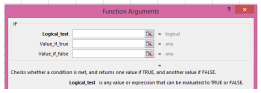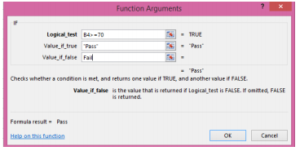# 4.6: IF function for Spreadsheets- Information

$$\newcommand{\vecs}{\overset { \rightharpoonup} {\mathbf{#1}} }$$ $$\newcommand{\vecd}{\overset{-\!-\!\rightharpoonup}{\vphantom{a}\smash {#1}}}$$$$\newcommand{\id}{\mathrm{id}}$$ $$\newcommand{\Span}{\mathrm{span}}$$ $$\newcommand{\kernel}{\mathrm{null}\,}$$ $$\newcommand{\range}{\mathrm{range}\,}$$ $$\newcommand{\RealPart}{\mathrm{Re}}$$ $$\newcommand{\ImaginaryPart}{\mathrm{Im}}$$ $$\newcommand{\Argument}{\mathrm{Arg}}$$ $$\newcommand{\norm}{\| #1 \|}$$ $$\newcommand{\inner}{\langle #1, #2 \rangle}$$ $$\newcommand{\Span}{\mathrm{span}}$$ $$\newcommand{\id}{\mathrm{id}}$$ $$\newcommand{\Span}{\mathrm{span}}$$ $$\newcommand{\kernel}{\mathrm{null}\,}$$ $$\newcommand{\range}{\mathrm{range}\,}$$ $$\newcommand{\RealPart}{\mathrm{Re}}$$ $$\newcommand{\ImaginaryPart}{\mathrm{Im}}$$ $$\newcommand{\Argument}{\mathrm{Arg}}$$ $$\newcommand{\norm}{\| #1 \|}$$ $$\newcommand{\inner}{\langle #1, #2 \rangle}$$ $$\newcommand{\Span}{\mathrm{span}}$$$$\newcommand{\AA}{\unicode[.8,0]{x212B}}$$

### OVERVIEW:

Where to find IF function: FORMULA tab, Logical in the Function group, then find IF in the pull down menu.

Purpose of IF function: To test for or evaluate specific conditions and then react differently depending on whether the test was TRUE or FALSE.

Return Value – the values you supply for TRUE or FALSE

Syntax: =IF(logical test, [value if true], [value if false])

Arguments:

• Logical test: A value or logical expression that can be evaluated as TRUE or FALSE
• Value if true: [optional] The value to return when logical test evaluates to TRUE.
• Value if false: [optional] The value to return when logical test evaluated to FALSE

EXERCISE (This isn’t graded, but you might find it useful before completing your assignment.)

If evaluating a student based on test scores, you want to assign either “Pass” or “Fail” to that student. You would be testing the score itself for each student and then returning either “Pass” or ”Fail”.1. Create a little spreadsheet like the one above with the headings Name, Score and Result. Type in five or six students and give them scores.

2. Click in cell C4 (in this example) to insert the formula result. Click on the FORMULA TAB, then click the Logical icon from the Function Library Group. Once you click on the Logical icon a menu appears with various Logical functions. Choose IF. The result is an argument box as seen below. Argument boxes are easier to work with because they show you where to put the information needed to complete the test.3. The first box (Logical Test) is asking for information for the test you want to perform. In this case, we want to set parameters on what is a passing grade. In that logical box, click cell B5 on the spreadsheet you created. Next put in the symbols (in the same box) that mean greater than an equal to (>=). This is followed by a grade of 70. (See other Logical Operators at the bottom of this page.)

4. In the next box (Value if true) type the word PASS without any quotation marks. The quotation marks will automatically be assigned to that text string when you click in the next box.

5. In the last box (Value if False), type the word FAIL without any quotation marks. The quotation marks will automatically be assigned to that text string when you click the OK button.

6. Below is what you will see if you followed these directions.7. Click OK. The formula now appears in cell C4 and on the formula bar.8. Pull the formula down for the other students and watch as either “Pass” or “Fail” appears for each student.

### Notes: More Logical Operators